# What is a Square Prism?

Brigette Banaszak, Joseph Vigil
• Author
Brigette Banaszak

Brigette has a BS in Elementary Education and an MS in Gifted and Talented Education, both from the University of Wisconsin. She has taught math in both elementary and middle school, and is certified to teach grades K-8.

• Instructor
Joseph Vigil

Joseph has a master's degree in literature as well as alternative teaching and ESL educator certifications. He has worked with middle school, high school, and college students in writing and language arts.

Learn about square prisms. Identify what a square prism is, discover how to find the volume and area of a square prism, and examine types of square-based prisms. Updated: 12/19/2021

Show

## What Is a Square Prism?

What is a prism? A prism is a three-dimensional solid with two congruent, parallel bases joined together by flat sides. A prism is named by the shape of its parallel bases, so a square prism is a prism with two congruent, parallel, square bases. A rectangular prism, on the other hand, has two congruent, parallel, rectangular bases. In the example, the square prism has two square bases (in red) and four rectangular sides (in yellow and blue). All square prisms are not cubes, although all cubes are square prisms.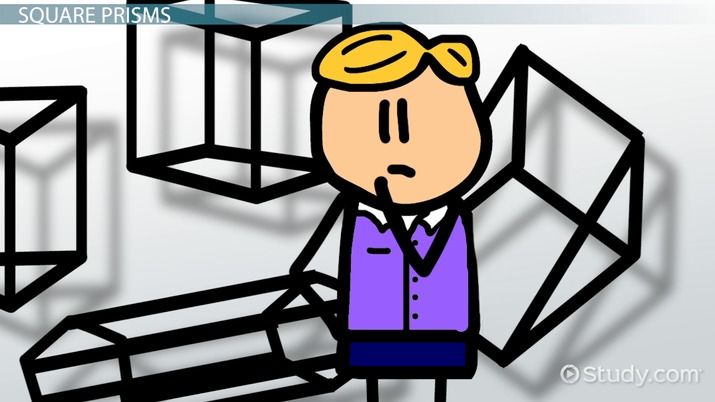An error occurred trying to load this video.

Try refreshing the page, or contact customer support.

Coming up next: Triangle Inequality: Theorem & Proofs

### You're on a roll. Keep up the good work!

Replay
Your next lesson will play in 10 seconds
• 0:05 Prisms
• 0:51 Square Prisms
• 2:19 Lesson Summary
Save Save

Want to watch this again later?

Timeline
Autoplay
Autoplay
Speed Speed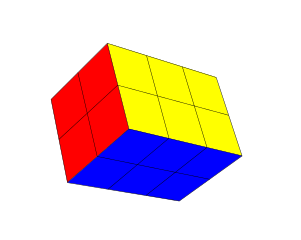## Properties of a Square Prism

What are the properties of a square prism? A square prism has:

• 6 faces: A face is a flat side on a three-dimensional solid shape. Opposite faces are congruent and parallel.
• 12 edges: An edge is the line segment where two faces meet.
• 8 vertices: The singular form of vertices is vertex. A vertex is a point, somewhat like a corner, where three or more edges meet.
• 1 set of congruent, parallel, square bases: A square prism can have more than two square bases, but there must be at least two.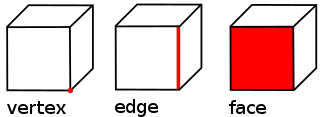The diagram shows several three-dimensional solids with edges and vertices labeled. There are two prisms, and one of them is a square prism. The 12 edges and 8 vertices on the square prism are marked.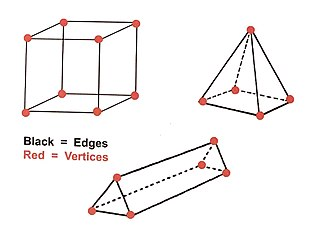The other two solids are a triangular prism, which has triangle bases, and a square pyramid. Notice how the pyramid has only one square base.

### Oblique vs. Right Square Prisms

There are two kinds of square prisms:

• A right square prism has sides that are perpendicular to its bases. This means the two bases will line up, one directly above the other.
• In an oblique square prism, the sides are not perpendicular to the prism's bases. Although the two bases will be congruent and parallel, one is not directly over the other.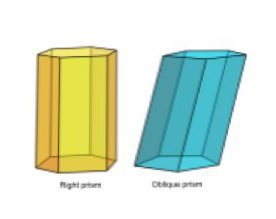The prisms shown in the diagram are hexagonal, not square, prisms, but they demonstrate the difference between a right prism and an oblique prism. The yellow prism on the left is a square prism, and the blue prism on the right is an oblique prism.

## Square Prism Examples

What are some real-world examples of square prisms?

A cube, such as these puzzle cubes, is an example of a square prism because it is a three-dimensional shape with at least two square sides.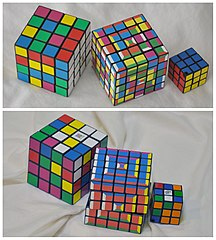This number cube is also a square prism.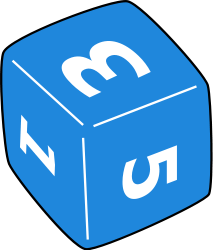This box of tissue is another example of a cube, which is a square prism.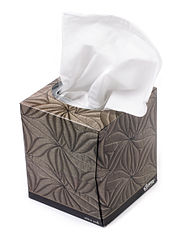These school lockers are square prisms because the bases of the lockers are squares.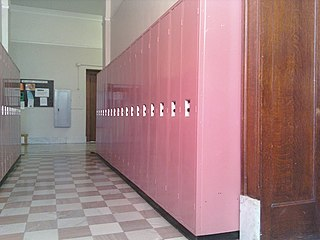A stack of several square crackers, one on top of the other, would make a square prism.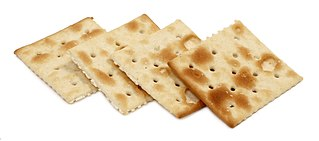A closed pizza box is a square prism.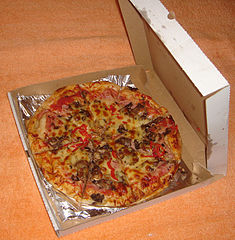In fact, any kind of box might be a square prism, whether used for moving, holding jewelry, or storing compact discs.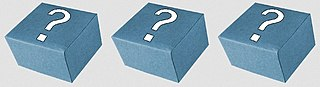Even a building can be a square prism, as long as the ground floor base is shaped like a square.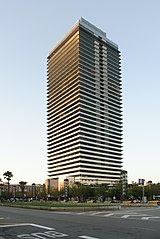### Net of a Square-Based Prism

A square prism is a three-dimensional shape. Three-dimensional shapes can be constructed from flat, two-dimensional nets.

The net of a right square-based prism will consist of at least two squares. The other sides may be either square or rectangles.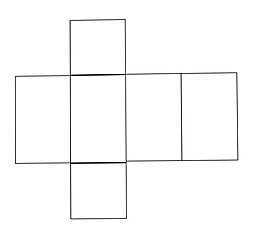The net of an oblique square-based prism will also consist of two squares, The other sides will be rhombuses or parallelograms.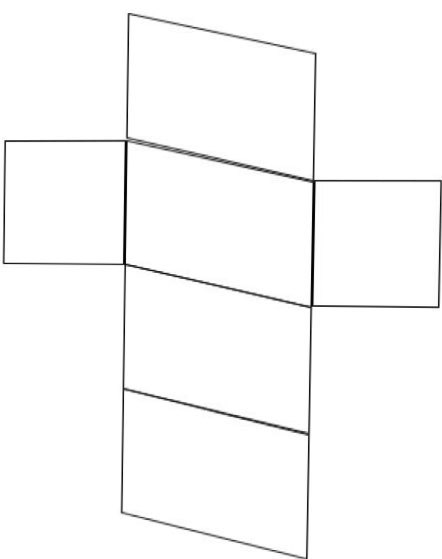## Square Prism Formulas

What is the area of a square prism? Since a square prism is a three-dimensional shape, it has a surface area rather than an area. The surface area of a square pyramid is the area of the outside of the prism. To find the surface area of a prism, find the sum of the areas of the prism's faces.

Look at the nets of the square prisms. Each net consists of two square bases, and either a large rectangle composed of four smaller rectangles or a large parallelogram composed of four smaller parallelograms. The large rectangle or parallelogram composed of four smaller shapes represents the sides of the prism. To find the surface area, find the area of the two square bases, and add to that total the area of the large shape composed of the lateral sides. That larger shape has a width that is equal to the perimeter of the base of the square prism, and a length that is equal to the height of the prism. So, the formula for the surface area of a square prism is:

To unlock this lesson you must be a Study.com Member.

#### What's the difference between a square prism and a rectangular prism?

A square prism has two congruent, parallel square bases, while a rectangular prism has two congruent, parallel rectangle bases. Every square prism is also a rectangular prism, since all squares are also rectangles.

#### How do you find the surface volume of a square prism?

To find the surface area of a square prism, find the sum of the areas of the prism's faces. The formula is Surface area = 2B + Ph, where B = the area of a square base, P = the perimeter of the square base, and h = the height of the prism.

#### Is a cube a prism?

A cube is an example of a square prism. A cube has two congruent, parallel, square bases, which makes it a square prism.

### Register to view this lesson

Are you a student or a teacher?

Back

### Resources created by teachers for teachers

Over 30,000 video lessons & teaching resources‐all in one place.Video lessonsQuizzes & WorksheetsClassroom IntegrationLesson Plans

I would definitely recommend Study.com to my colleagues. It’s like a teacher waved a magic wand and did the work for me. I feel like it’s a lifeline.

Jennifer B.
TeacherCreate an account to start this course today
Used by over 30 million students worldwide